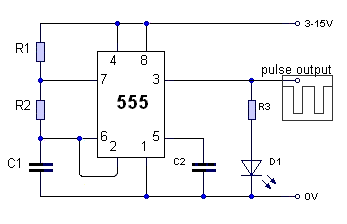555 - AstableR1 =   K R2 =   K C1 =   μF Calculate the astable frequency (f) to 3 sig figs
 Astable (pulse output) frequency (f) =  Hz
For more detail look here
The formula is in the specification. Also, click on 'Help'.
It will be given in the examination.
You will need a calculator!

home